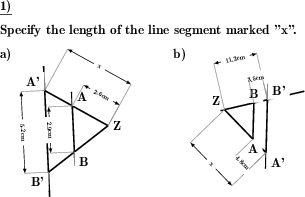Custom math worksheets at your fingertipsDetails for problem "Determine line segment length with intercept theorem"

Quickname: 8979

Junior High School, Middle School, High School.

Summary

In application of the intercept theorem the fourth line segment length is to be calculated from three given lengths.

ExamplesDescription

A labeled drawing to illustrate the intercept theorems is shown. On the figure three lines are labeled with their length. The inscription does not correspond to the actual length. Therefore no lengths are to be determined by measurement. However, the relations of the line segments to each other are correct.

The task is to calculate the length of the fourth line segment, which is labeled with the letter "x".

One of the intercept theorems is to be used for this. If desired, a reference to the intercept theorems can also be included in the problem definition.

To do so the following three steps are necessary

1. Set up the equation of proportion according to the radiation theorems.
2. Solve the equation for x
3. Calculate the solution.

These steps are shown in the solution. The problem can be set so that these steps are also given in the assignment.

Examples:

| ZA | / | ZA' | = | ZB | / | ZB' |
x / 5 = 7 / 14
x = 7 * 5 / 14

x = 2.5

Which theorems are tested is configurable. The figure is presented according to the queried ray-set: For the first and second theorem the figure has two straight lines or rays, for the third theorem three. The figure can be drawn in V-shape, X-shape, or in a random selection of both variants.

The inscription of the points can be optionally

• fixed to Z for the intersection of the lines, A and A' for the points on one line and B and B' for the points on the other line.
• random for the intersection, and random pairs of X and X' for both lines.
• completely random, each point is labeled individually.

The size of the drawing can be selected in several steps. The number of problems can also be configured.

Download free printable worksheets for this math problem here. The worksheet contains the problems only, the solution sheet includes the answers. Just click on the respective link.

•Worksheet 1Solution sheet with answers
•Worksheet 2Solution sheet with answers
•Worksheet 3Solution sheet with answers

If you can not see the solution sheets for download, they may be filtered out by an ad blocker that you may have installed. If this is the case, please allow ads for this page and reload the page. The solution sheets will then reappear.

• Do these sample worksheets do not really fit?
• Do you need more math worksheets, with a different level of difficulty?
• Would you like to combine different problems on a worksheet and adjust them to your needs?
• As a teacher, you can put together your own worksheets using the automatically generated math problems provided.
With a free initial credit, you can start creating your own math worksheets in a few minutes.

You can try it for free! Register here, to create custom worksheets now!

Customization options for this problem

Parameter
Possible values
Number of problems
1, 2, 3, 4
Size of drawing
small, medium, large
Point names
Z,A,A',B,B', Pairs with ', All different
Theorems covered
First, second, third, First, Second, Third, First & second, First & third, Second & third
Type of drawing
V or X, V, X
Mention intercept theorems
No reference, General reference, Show calculation steps

Similar problems

Remark
Description
Without actually calculating any lengths
In a statement of the intercept theorems the missing line segment name has to be inserted.
Knowledge of the rules, i.e. the equations of ratios
Correct statements on the intercept theorems must be identified and marked.Deutsche Version dieser Aufgabe
These informational pages with samples describe math problems that can be combined on custom math worksheets with solutions for home and K-12 school use.
Deutsche Seiten
×